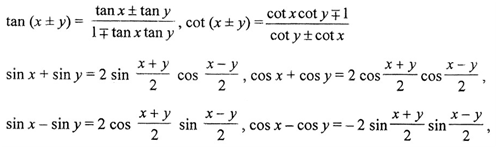## Mathematics 1st Year CHSE syllabus

Mathematics 2021-22

1st Year Revised  (CHSE)    (SC )

UNIT – I : Sets and Functions

1. 1. Sets

Sets and their representations. Empty set, Finite and Infinite sets, Equal sets, Subsets, Subsets of a set of real numbers especially intervals (with notations), Power set, Universal set, Venn diagrams, Union and Intersection of sets, Difference of sets, complement of a set, Properties of Complement of Sets, Practical Problems based on sets.

1. Relations & Functions

Ordered pairs, Cartesian product of sets. Number of elements in the Cartesian product of two finite sets. Cartesian product of the sets of real (up to R × R). Definition of relation, pictorial diagrams, domain, co-domain and range of a relation. Function as a special kind of relation from one set to another. Pictorial representation of a function, domain co-domain and range of a function. Real valued functions, domain and range of these functions: Constant, identity, polynomial, rational, Modulus, signum, exponential, logarithmic and greatest integer function, with their graphs.

1. Trigonometric Functions

Positive and negative angles. Measuring angles in radians and in degrees and conversion of one into other. Definition of trigonometric functions with the help of unit circle. Truth of sin2x + cos2x = 1, for all x. Signs of trigonometric functions. Domain and range of trigonometric functions and their graphs. Expressing sin (x ± y) and cos (X± y) in terms of sinx, siny, cosx& cosy and their simple application. Deducing identities like the following :Identities related to sin 2x, cos 2x, tan 2x, sin 3x, cos 3x and tan 3x. Trigonometric equations Principal solution.

UNIT-II : Algebra

1. Principle of Mathematical Induction

Process of the proof by induction, motivation the application of the method by looking at natural numbers as the least inductive subset of real numbers. The principle of Mathematical induction and simple applications.

1. Complex Numbers and Quadratic Equations

Need for complex numbers, especially 1, to be motivated by inability to solve some of the quadratic equations; Algebraic properties of complex numbers. Argand plane. Statement of Fundamental Theorem of Algebra, solution of quadratic equations in the complex system. cube roots of unity and its properties.

1. Linear Inequalities

Linear inequalities. Algebraic solutions of linear inequalities in one variable and their representation on the number line. Graphical solution of linear inequalities in two variables. Graphical solution of system of linear inequalities in two variables.

1. Permutations and Combinations

Fundamental principle of counting, factorial n. (n!), Permutations and combinations, simple applications.

1. Binomial Theorem

History, statement No problems on Binomial Theorem

1. Sequence and Series

Sequence and Series, Arithmetic Progression (A.P.). Arithmetic Mean (A.M.) Geometric Progression (G.P.), general term of a G.P, sum of n terms of a G.P., Arithmetic and Geometric series, infinite G.P. and its sum, geometric mean (G.M.), Harmonic (mean) relation between A.M., GM. and H.M.,

UNIT – III : Co-ordinate Geometry

1. Straight Lines

Brief recall of two dimensional geometry from earlier classes. Slope of a line and angle between two lines. Various forms of equations of a line : parallel to axis, point-slope form, slope-intercept form, two-point form, intercept form and normal form. General equation of a line. Equation of family of lines passing through the point of intersection of two lines. Distance of a point from a line,.

1. Conic Sections

Sections of a cone : circles, ellipse, parabola, hyperbola; Standard equations and simple properties of Circle, parabola, ellipse and hyperbola.

1. Introduction to Three-dimensional Geometry

Coordinate axes and coordinate planes in three dimensions. Coordinates of a point. Distance between two points and section formula.

UNIT-IV: Calculus

1. Limits and Derivatives

Derivative introduced as rate of change both as that of distance function and geometrically. Intuitive idea of limit. Limits of polynomials and rational functions, trigonometric, exponential and logarithmic functions. Definition of derivative, relate it to slope of tangent of a curve, derivative of sum, difference, product and quotient of functions. The derivative of polynomial and trigonometric functions.

UNIT-V : Mathematical Reasoning

1. Mathematical Reasoning

Mathematically acceptable statements. Connecting words/phrases-consolidating the understanding of “if and only if (necessary and sufficient) condition,” “implies”, “and/ or”, “implied by”, “and”, “or”, “there exists” and their use through variety of examples related to real life and Mathematics.

UNIT-VI : Statistics and Probability

1. Statistics

Measures of dispersion; Range, mean deviation, variance and standard deviation of ungrouped/ grouped data.

Random experiments; outcomes, sample spaces (set representation). Events; occurrence of events, ‘not’, ‘and’ and ‘or’ events, exhaustive events, mutually exclusive events, Probability of an event. Probability of ‘not’, ‘and’ ‘or’ events.

[ Books Recommended:

Bureau’s Higher Secondary (+2) Elements of Mathematics, Part-I, Published by Odisha State Bureau of Text Book Preparation and Production, Bhubaneswar.]

— ALL THE BEST —